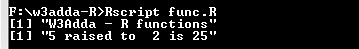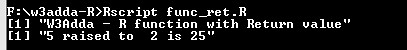# R Function

In this tutorial you will learn about the R Function and its application with practical example.

## R Function

Function gives you way to wrap up the set of statements that means to perform any specific task and give it a name, so that it can be invoked later from any where in the program. Functions makes it easy to divide the complete program into sub-units that perform a specific task for that program, this way it enhance the modular approach and increase the code re-usability of the program. We pass information in function call as its parameter and the function can either returns some value to the point it where it called from or returns nothing.

## Advantages of function

• It enhance the modularity of the program.
• It enhance the re usability.
• It makes development easy as development can be shared in team.
• It reduces the coupling.
• It reduces duplication.

## Type Of Function In R

• Built in function
• User defined functions

## R Built In Function

Built in functions are the functions available in R to perform some common and standard tasks. R supports a rich set of in-built functions including file access, mathematical computations, graphics, memory management etc.

## R User defined functions

User defined function are custom function defined by user it self to perform as custom task that is not available as built in, in this way user can define and write subprograms as functions to perform a task relevant to their programs.

## Defining a function In R

In R, a function can be declared using “function” keyword with list of required parameters if any as following –

Syntax:-

Above is the general syntax of a R function, here

function :- It is R keyword which is used to define a function.
func_name :- It is replaced with the name of the function.
parameter_list :- It represents the list of the parameters need to be passed when function call made.

Example:-

Let’s create a function to calculate the power of given number.

## Calling a function In R

In R, a function can be invoked simply by its name with arguments list as following –

let’s put together in R script as following –

Example:-

When run the above R script, we see the output as following –

Output:-## Return Value from Function

Sometimes we may want a function to return some value to the point it where it is called from. In R, there is return() function allows a function to return value.

Syntax:-

Example:-

Let modify the above function we created to return power of given number.

Output:-## R Function with Multiple Return Values

In R, if we want a function to return multiple values, this can be achieved using a list object in return() as following –

Example:-

Output:-In this tutorial we have learn about the R Function and its application with practical example. I hope you will like this tutorial.